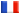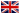# Séminaire de Cryptographie

## Ralf Gerkmann### Relative rigid cohomology and the deformation method for elliptic curves

By a well-known result of Dwork the zeta functions of the fibers in a one-parameter family of hypersurfaces can be described in terms of p-adic holomorphic functions. This result was used by A. Lauder in order to formulate a deter- ministic algorithm that computes the zeta function of a hypersurface in polynomial time. In this talk we describe a similiar method for elliptic curves which is based on rigid cohomology rather than Dwork cohomology. In contrast to Dwork's theory, rigid cohomology is closely related to the notions of classical algebraic geometry. We give an overview on the theoretical background, describe the essential steps of the algorithm and comment on the problem of p-adic precision estimates. We also report on computational results obtained by a MAGMA implementation. In the last part we explain the relation between both theories and how Lauder's general algorithm can be reformulated in terms of rigid cohomology. This shows up similiarities as well as differences between the two approaches.